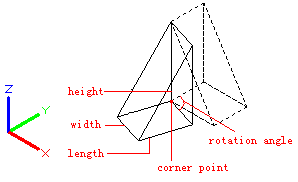# 3D command

## The 3D command is used to create 3D polyface meshes with common geometric shapes.

Command

3D

Command Prompts

Enter an option [ Box/Cone/DIsh/DOme/Mesh/Pyramid/Sphere/Torus/Wedge ]:

Relative Glossary:

Box :
Create a 3D box polyface mesh.

• Corner point of box: Specify corner point of box mesh.
• Length of box: Specify length of box mesh.
• Width of box: Specify width of box mesh by inputting a value or specifying a point.
• Height of box: Specify height of box mesh by inputting a value or specifying a point.
• Rotation angle of box: Specify rotation angle of box mesh. The box mesh is rotated around the specified corner point; if inputting 0, the box mesh will be orthogonal to X axis and Y axis of current UCS.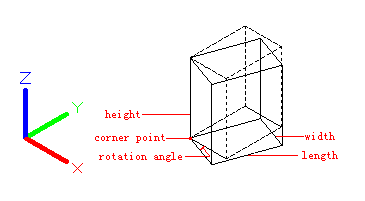Reference:
Align the box mesh to other object in drawing or rotate it by a specified angle. The rotation base point is the specified corner point of box mesh.

Cube:
Create a cube with equal length, width and height.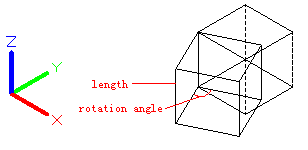Cone:
Create a cone-shaped polygon mesh.

• Radius / Diameter for base: Define the cone base by its radius or diameter.
• Radius / Diameter for top: Define the cone top by its radius or diameter. If the radius or diameter is 0, it will create a cone; if the radius or diameter is larger than 0, it will create a truncated cone.
• Height: Specify cone height.
• Number: Specify number of segments for surface of cone.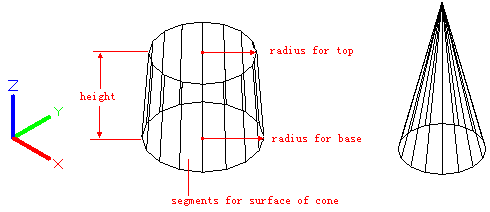DIsh:
Create the lower part of spherical polygonal mesh.

• Radius / Diameter of dish: Define a dish by its radius or diameter.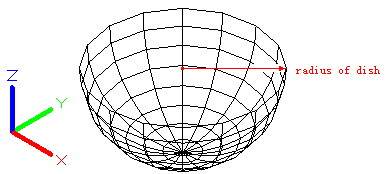DOme:
Create the top part of spherical polygonal mesh.

• Radius / Diameter of dome: Define a dome by its radius or diameter.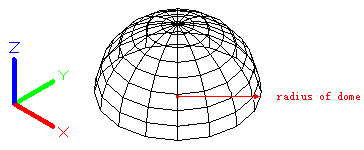Mesh:
Create a planar mesh; its sizes in M direction and N direction determine number of lines. The M direction and N direction are similar to X axis and Y axis in XY plane.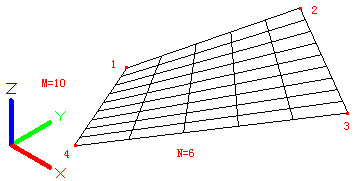Pyramid:
Create a pyramid or a tetrahedron mesh.

• Corner point: Define four corner points of a pyramid. The Z value of specified point determines the vertex, top surface, or ridge of pyramid.
• Apex point: Define the top of pyramid as a point (apex point).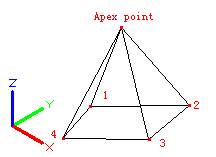• Ridge: Define the top of pyramid as a ridge. The order of two endpoints should be the same as base points to avoid self-intersection.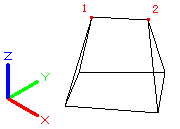• Top: Define the top of pyramid as a quadrangle.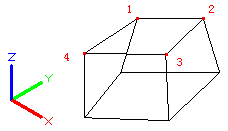Sphere:
Create a spherical polygon mesh.

• Radius / Diameter: Define a sphere by its radius or diameter.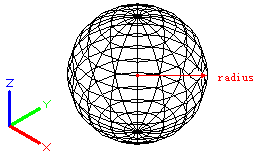Torus:
Create a thyroidal polygon mesh. It is parallel to the XY plane of the current UCS.
The radius of torus is from the center to the outmost rather than the torus center to the tube center.

• Radius / Diameter of torus: Define a torus by its radius or diameter.
• Radius / Diameter of tube: Define a tube by its radius or diameter. The radius is from the center of tube to the outmost.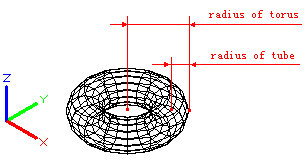Wedge:
Create a right-angle, wedge-shaped polygon mesh. The sloped face is tapering along the X axis.
The rotation base point is the corner point of wedge. Inputting 0 as rotation angle, the wedge mesh will be orthogonal to X axis and Y axis of current UCS.